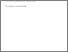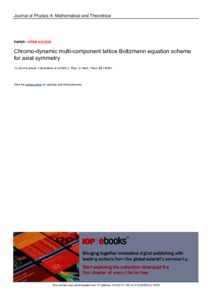# Chromo-dynamic multi-component lattice Boltzmann equation scheme for axial symmetry

SPENDLOVE, James Edward, XU, Xu, SCHENKEL, Torsten, SEATON, Michael Andrew and HALLIDAY, Ian (2020). Chromo-dynamic multi-component lattice Boltzmann equation scheme for axial symmetry. Journal of Physics A: Mathematical and Theoretical, 53 (14), p. 145001.Preview
PDF
XU-ChromoDynamicMulti(VoR).pdf - Published Version

Open Access URL: https://iopscience.iop.org/article/10.1088/1751-81... (Published)

## Abstract

We validate the chromo-dynamic multi-component lattice Boltzmann equation (MCLBE) simulation for immiscible fluids with a density contrast against analytical results for complex flow geometries, with particular emphasis on the fundamentals of the method, i.e. compliance with inter-facial boundary conditions of continuum hydrodynamics. To achieve the necessary regimes for the chosen validations, we develop, from a three-dimensional, axially-symmetric flow formulation, a novel, two-dimensional, pseudo Cartesian, MCLBE scheme. This requires the inclusion in lattice Boltzmann methodology of a continuously distributed source and a velocity-dependent force density (here, the metric force terms of the cylindrical Navier-Stokes equations). Specifically, we apply our model to the problem of flow past a spherical liquid drop in Re=$0$, Ca$\rightarrow 0$ regime and, also, flow past a lightly deformed drop. The resulting simulation data, once corrected for the simulation's inter-facial micro-current (using a method we also advance herein, based on freezing the phase field) show good agreement with theory over a small range of density contrasts. In particular, our data extend verified compliance with the kinematic condition from flat \cite{Burgin} to the case of curved fluid-fluid interfaces. More generally, our results indicate a route to eliminate the influence of the inter-facial micro-current.

Item Type: Article 01 Mathematical Sciences; 02 Physical Sciences; Mathematical Physics https://doi.org/10.1088/1751-8121/ab777f p. 145001 Symplectic Elements Symplectic Elements 26 Feb 2020 12:55 18 Mar 2021 02:16 https://shura.shu.ac.uk/id/eprint/25890View Item